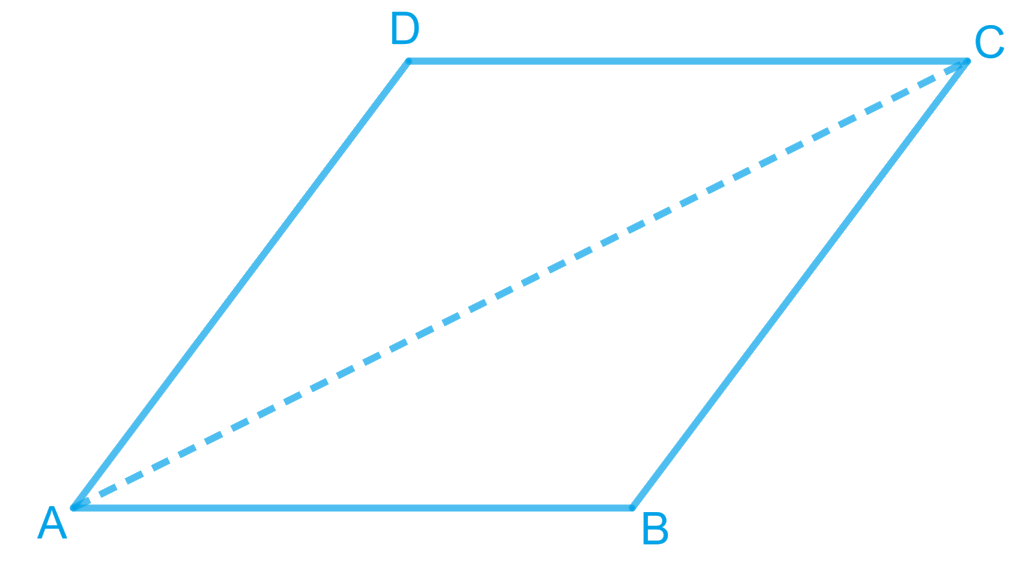# Ex.8.1 Q7 Quadrilaterals Solution - NCERT Maths Class 9

Go back to  'Ex.8.1'

## Question

$$ABCD$$ is a rhombus. Show that diagonal $$AC$$ bisects $$\angle A$$ as well as $$\angle C$$ and diagonal $$BD$$ bisects $$\angle B$$ as well as $$\angle D$$.

Video Solution
Ex 8.1 | Question 7

## Text Solution

What is known?

ABCD is a rhombus.

What is unknown?

How we can show that diagonal $$AC$$ bisects $$∠A$$ as well as $$∠C$$ and diagonal BD bisects $$∠B$$ as well as $$∠D.$$

Reasoning:

We can use alternate interior angles property to show diagonal $$AC$$ bisects angles $$A$$ and $$C$$, similarly diagonal $$BD$$ bisects angles B and D.

Steps:Let us join $$AC$$.

In $$\Delta$$$$ABC$$,

\begin{align}&BC=AB\\&\left( \begin{array} & \text{Sides of a rhombus are} \\ \text{ equal to each other} \\ \end{array} \right)\\\\&\angle1=\angle2\\&\left( \begin{array} & \text{Angles opposite to equal sides } \\ \text{ of a triangle are equal} \\ \end{array} \right)\end{align}

However, $$\angle 1 = \angle 3$$ (Alternate interior angles for parallel lines $$AB$$ and $$CD$$)

$$\angle 2 = \angle 3$$

Therefore, $$AC$$ bisects $$\angle C$$.

Also, $$\angle 2 = \angle 4$$ (Alternate interior angles for || lines $$BC$$ and $$DA$$) $$\angle 1 = \angle 4$$

Therefore, $$AC$$ bisects $$\angle A$$.

Similarly, it can be proved that $$BD$$ bisects $$\angle B$$ and $$\angle D$$ as well.

Video Solution